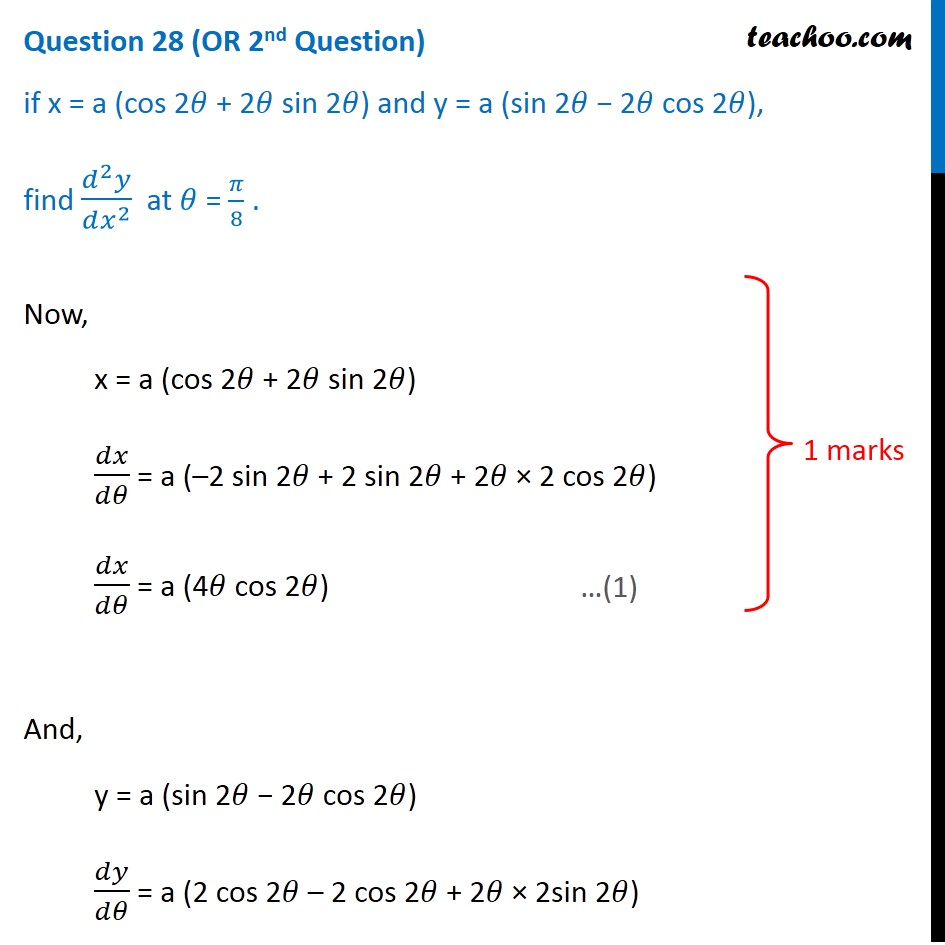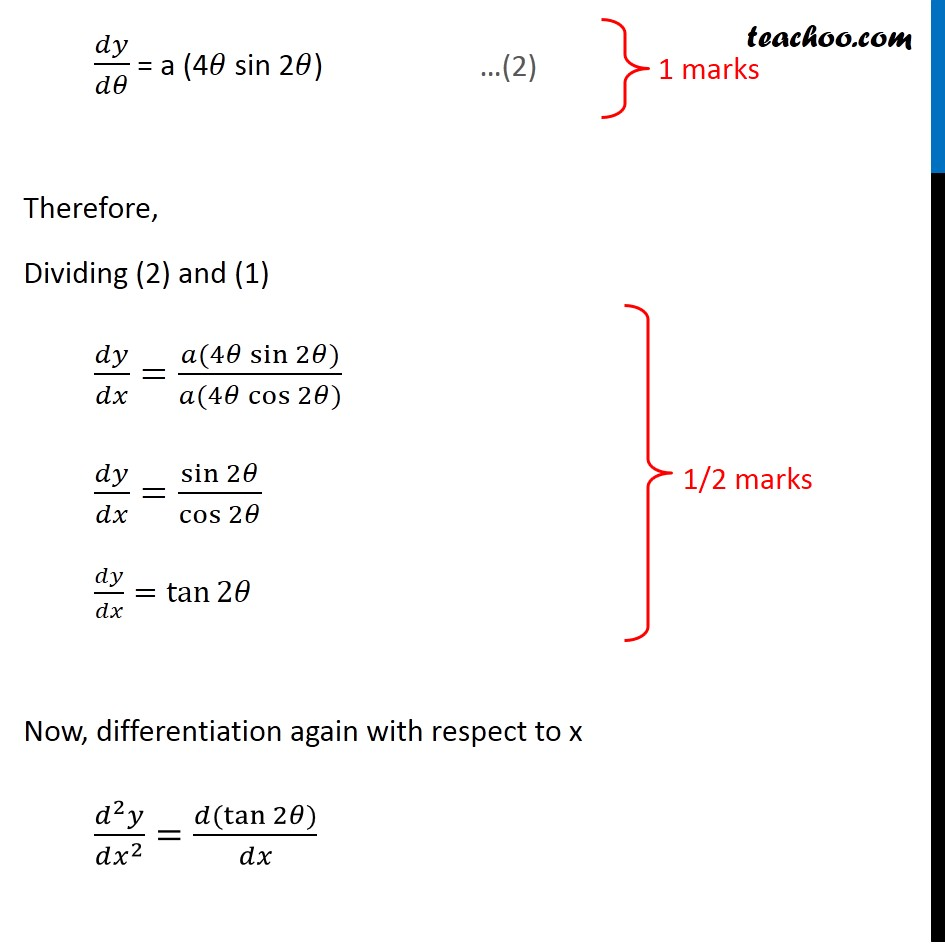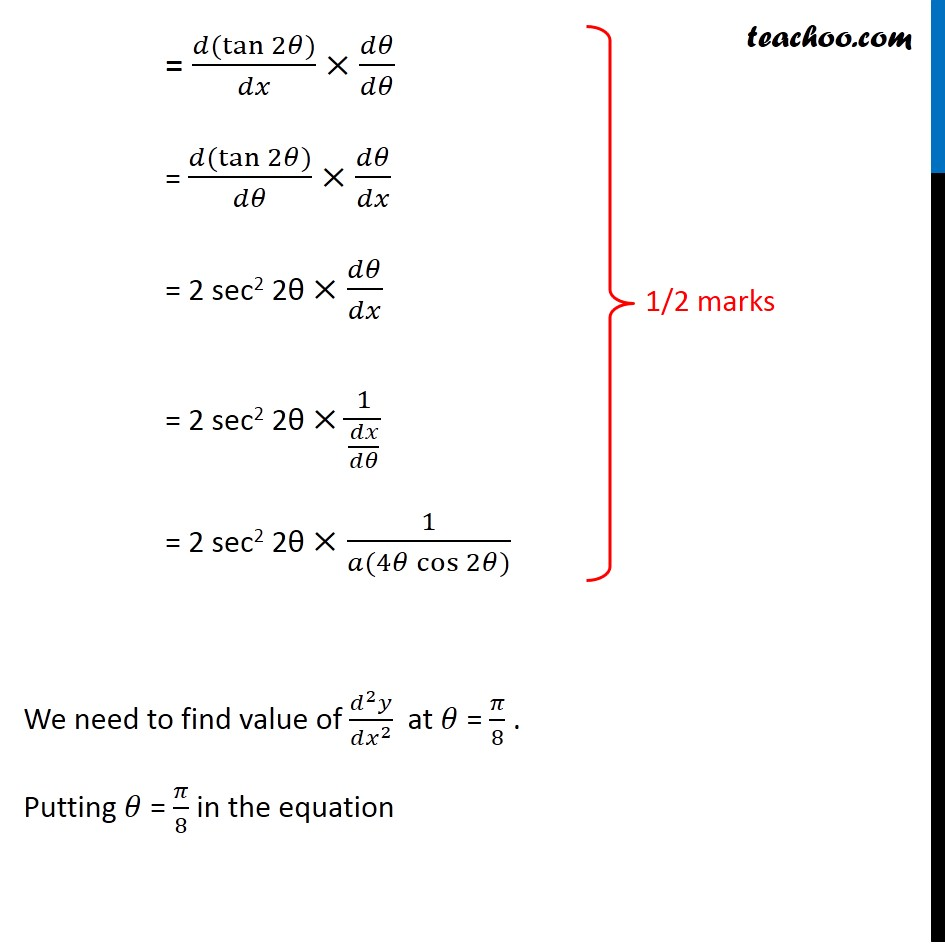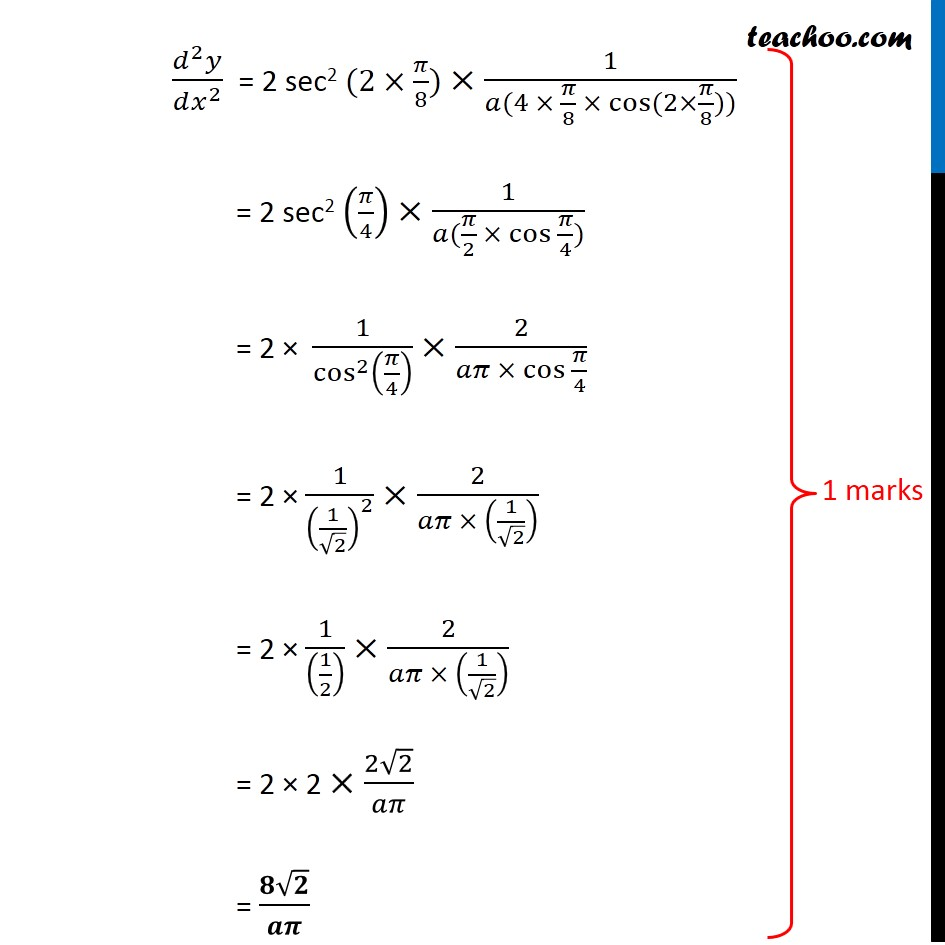## find (d 2 y)/(dx 2 )  at θ = π/8 .Subscribe to our Youtube Channel - https://you.tube/teachoo

1. Class 12
2. Solutions of Sample Papers and Past Year Papers - for Class 12 Boards
3. CBSE Class 12 Sample Paper for 2020 Boards

Transcript

Question 28 (OR 2nd Question) if x = a (cos 2𝜃 + 2𝜃 sin 2𝜃) and y = a (sin 2𝜃 − 2𝜃 cos 2𝜃), find (𝑑^2 𝑦)/(𝑑𝑥^2 ) at 𝜃 = 𝜋/8 . Now, x = a (cos 2𝜃 + 2𝜃 sin 2𝜃) 𝑑𝑥/𝑑𝜃 = a (–2 sin 2𝜃 + 2 sin 2𝜃 + 2𝜃 × 2 cos 2𝜃) 𝑑𝑥/𝑑𝜃 = a (4𝜃 cos 2𝜃) And, y = a (sin 2𝜃 − 2𝜃 cos 2𝜃) 𝑑𝑦/𝑑𝜃 = a (2 cos 2𝜃 – 2 cos 2𝜃 + 2𝜃 × 2sin 2𝜃) 𝑑𝑦/𝑑𝜃 = a (4𝜃 sin 2𝜃) Therefore, Dividing (2) and (1) 𝑑𝑦/𝑑𝑥=(𝑎(4𝜃 sin⁡2𝜃))/(𝑎(4𝜃 cos⁡2𝜃)) 𝑑𝑦/𝑑𝑥=sin⁡2𝜃/cos⁡2𝜃 𝑑𝑦/𝑑𝑥=tan⁡2𝜃 Now, differentiation again with respect to x (𝑑^2 𝑦)/(𝑑𝑥^2 )=(𝑑(tan⁡2𝜃))/𝑑𝑥 = (𝑑(tan⁡2𝜃))/𝑑𝑥×𝑑𝜃/𝑑𝜃 = (𝑑(tan⁡2𝜃))/𝑑𝜃×𝑑𝜃/𝑑𝑥 = 2 sec2 2θ ×𝑑𝜃/𝑑𝑥 = 2 sec2 2θ ×1/(𝑑𝑥/𝑑𝜃) = 2 sec2 2θ ×1/(𝑎(4𝜃 cos⁡2𝜃)) We need to find value of (𝑑^2 𝑦)/(𝑑𝑥^2 ) at 𝜃 = 𝜋/8 . Putting 𝜃 = 𝜋/8 in the equation (𝑑^2 𝑦)/(𝑑𝑥^2 ) = 2 sec2 (2×𝜋/8) ×1/(𝑎(4 × 𝜋/8 × cos⁡〖(2×𝜋/8)〗)) = 2 sec2 (𝜋/4) ×1/(𝑎(𝜋/2 × 〖cos 〗⁡〖𝜋/4〗)) = 2 × 1/cos^2⁡(𝜋/4) ×2/(𝑎𝜋 × 〖cos 〗⁡〖𝜋/4〗 ) = 2 × 1/(1/√2)^2 ×2/(𝑎𝜋 × (1/√2) ) = 2 × 1/((1/2) ) ×2/(𝑎𝜋 × (1/√2) ) = 2 × 2 ×(2√2)/𝑎𝜋 = (𝟖√𝟐)/𝒂𝝅

CBSE Class 12 Sample Paper for 2020 Boards

Class 12
Solutions of Sample Papers and Past Year Papers - for Class 12 Boards